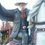# Divisors of 999...9999 in the "right way"

I studied something after making that crazy thing. This time I will use the Euler's theorem which states that, if two positive integers $a$ and $b$ are relatively prime to themselves, then:

$a^{\phi(b)}\equiv1\bmod {b}$

Where $\phi(b)$ is the Euler's totient function, which returns the size of the set $\mathbb{S}$, where $\mathbb{S}=\{x\in\mathbb{N}|\text{gcd}(b, x)=1\text{ and }x

This is true for $a=10$ and $b$ is a number that it is not a multiple of 2, nor 5. Therefore:

$b\mid 10^{\phi(b)}-1=999....999$

I should alert anyone reading this that $\phi(b)$ does not need to be the smallest positive integer that will make this work, let $b=3$:

$3|10^{\phi(3)}-1=10^2-1=99$

But:

$3|9=10^1-1$

However, we can expand this concept to sequences of other numbers; Writing this in another way, calling $\phi(b)=n$ because I already showed that a "$n$" exists under what conditions:

$kb=10^n-1, k\in\mathbb{N}^*$

I will multiply this by $s=1+10^{n}+10^{2n}+...+10^{8n}=\sum^8_{k=0}10^{kn}$, notice that s is a multiple of 9; This can be proved this way:

$\sum^8_{k=0}10^{kn}\equiv\sum^8_{k=0}(10-9)^{kn}\equiv\sum^8_{k=0}1^{kn}\equiv\sum^8_{k=0}1\equiv9\equiv1\bmod{9}$

Therefore $s=9t, t\in\mathbb{N}^*$

Multiplying both the two equations and using telescopic sums:

$skb=(10^n-1)\times\sum^8_{k=0}10^{kn}$

$skb=10^n\times\sum^8_{k=0}10^{kn}-\sum^8_{k=0}10^{kn}$

$skb=\sum^9_{k=1}10^{kn}-\sum^8_{k=0}10^{kn}$

$skb=\sum^9_{k=1}10^{kn}-\sum^8_{k=0}10^{kn}$

$skb=10^{9n}-1=999...999$

Remeber that $s=9t$

$9tkb=999...999$

$tkb=111...111$

Since $t, k \in\mathbb{N}^*$, $tk\in\mathbb{N}^*$; Therefore $(\forall b\in\{x\in\mathbb{N})(2\nmid b \wedge 5\nmid b\}\exists f,n\in\mathbb{N}^* | fb=\sum^{n}_{k=0}10^k)$

Just to make this complete, if $2\mid b$ or $5\mid b$, let $b=2^\alpha5^\beta c, 2\nmid c, 5\nmid c, c\in\mathbb{N}^*$, $\gamma=\max(\alpha,\beta)$

Multiply everything by $z=10^\gamma2^{-\alpha}5^{-\beta}$, note that this number is natural;

$bz=10^{\gamma}c$

Note that $c$ meets the conditions that allow me do this:

$kc=\sum^n_{k=0}10^k$

Multiplying by k the first equation:

$bzk=10^\gamma\sum^n_{k=0}10^k=\sum^n_{k=0}10^{k+\gamma}=\sum^{n+\gamma}_{k=\gamma}10^k$

This means I can generalize my result:

$\forall b\in\mathbb{N}^*\exists l, m, n\in\mathbb{N}|bl=\sum^{m}_{k=n}10^k$

I can generalize even more, using another base system:

Remember the Euler's theorem if a and b are co-prime :

$a^{\phi(b)}\equiv1\bmod {b}$

Rewriting, with $\phi(b)=n$:

$bk=a^n-1= \overline{(a-1)(a-1)(a-1)....(a-1)(a-1)(a-1)}$

Imagine that each (a-1) is a digit;

I will multiply everything by $c=\sum^{a-2}_{k=0}a^{nk}$, but first I will prove that this a multiple of $a-1$

$\sum^{a-2}_{k=0}a^{nk}\equiv\sum^{a-2}_{k=0}(a-(a-1))^{nk}\equiv\sum^{a-2}_{k=0}1^{nk}\equiv\sum^{a-2}_{k=0}1\equiv a-1\equiv 0\bmod {a-1}$

$bck=(a^n-1)\sum^{a-2}_{k=0}a^{nk}$

$bck=a^n\sum^{a-2}_{k=0}a^{nk}-\sum^{a-2}_{k=0}a^{nk}$

$bck=\sum^{a-1}_{k=1}a^{nk}-\sum^{a-2}_{k=0}a^{nk}$

$bck=a^{n(a-1)}-1=\overline{(a-1)(a-1)(a-1)....(a-1)(a-1)}$

As I proved before $c=d(a-1), d\in\mathbb{N}^*$

$(a-1)bdk=\overline{(a-1)(a-1)(a-1)....(a-1)(a-1)}$

$bdk=(111...111)_a$

Since $dk\in\mathbb{N}^*$, I can say:

$(\forall b, a\in\mathbb{N}^*)(\text{gcd}(a,b)=1\Leftrightarrow\exists n, f|bf=\sum^n_{k=0} a^k)$

The other way can be proven by observing:

$bf\equiv1\mod a$

This means $bf-ka=1, k\in\mathbb{N}$, with this in mind, I will prove that $\text{gcd}(a,b)=L$ must be 1:

$b'L=b, a'L=a$

$a',b'\in\mathbb{N}$

Doing a substitution

$b'Lf-a'Lk=1$

$L(b'f-a'k)=1$

As both of the factors are positive integers, both of them are 1

Now I will study the case where $\text{gcd}(a,b)\neq1$

I will consider the prime factorization of $a$:

$a=\prod^z_{j=1}p_j^{a_j}$

$p_j$ is the $j$-th prime, $a_j$ is its exponent, $z$ is the largest number that makes $a_z\neq0$

I will make $b=c\prod^n_{j=1}p_j^{b_j}$, this time $(\forall j)(a_j\neq0\Leftrightarrow p_j\nmid c)\wedge(a_j=0\Rightarrow b_j=0)$

Let $\gamma=\max(\{x\in\mathbb{N}^*|x=\left\lceil\frac{b_j}{a_j}\right\rceil j\in\mathbb{N}\wedge a_j\neq0\})$

I will multiply everything by $d=a^\gamma\prod^n_{j=1}p_j^{-b_j}$, notice that this number is a natural number

$bd=a^\gamma c$

Since $\text{gcd}(a,c)=1$, I can say that

$ck=\sum^n_{j=0}a^j$

Multiplying k on every side of the other equation:

$bdk=a^\gamma ck$

Substituting $ck$:

$bdk=a^\gamma \sum^n_{j=0}a^j$

$bdk=a^\gamma \sum^n_{j=0}a^{j+\gamma}$

$bdk=\sum^{n+\gamma}_{j=\gamma}a^j$

Since $dk\in\mathbb{N}^*$ I can say that(finally):

$(\forall a,b\in\mathbb{N}^*)(\exists k,m,n\in\mathbb{N}^*)(bk=\sum^m_{j=n}a^j)$Note by Matheus Jahnke
4 years, 4 months ago

This discussion board is a place to discuss our Daily Challenges and the math and science related to those challenges. Explanations are more than just a solution — they should explain the steps and thinking strategies that you used to obtain the solution. Comments should further the discussion of math and science.

When posting on Brilliant:

• Use the emojis to react to an explanation, whether you're congratulating a job well done , or just really confused .
• Ask specific questions about the challenge or the steps in somebody's explanation. Well-posed questions can add a lot to the discussion, but posting "I don't understand!" doesn't help anyone.
• Try to contribute something new to the discussion, whether it is an extension, generalization or other idea related to the challenge.

MarkdownAppears as
*italics* or _italics_ italics
**bold** or __bold__ bold
- bulleted- list
• bulleted
• list
1. numbered2. list
1. numbered
2. list
Note: you must add a full line of space before and after lists for them to show up correctly
paragraph 1paragraph 2

paragraph 1

paragraph 2

[example link](https://brilliant.org)example link
> This is a quote
This is a quote
    # I indented these lines
# 4 spaces, and now they show
# up as a code block.

print "hello world"
# I indented these lines
# 4 spaces, and now they show
# up as a code block.

print "hello world"
MathAppears as
Remember to wrap math in $$ ... $$ or $ ... $ to ensure proper formatting.
2 \times 3 $2 \times 3$
2^{34} $2^{34}$
a_{i-1} $a_{i-1}$
\frac{2}{3} $\frac{2}{3}$
\sqrt{2} $\sqrt{2}$
\sum_{i=1}^3 $\sum_{i=1}^3$
\sin \theta $\sin \theta$
\boxed{123} $\boxed{123}$